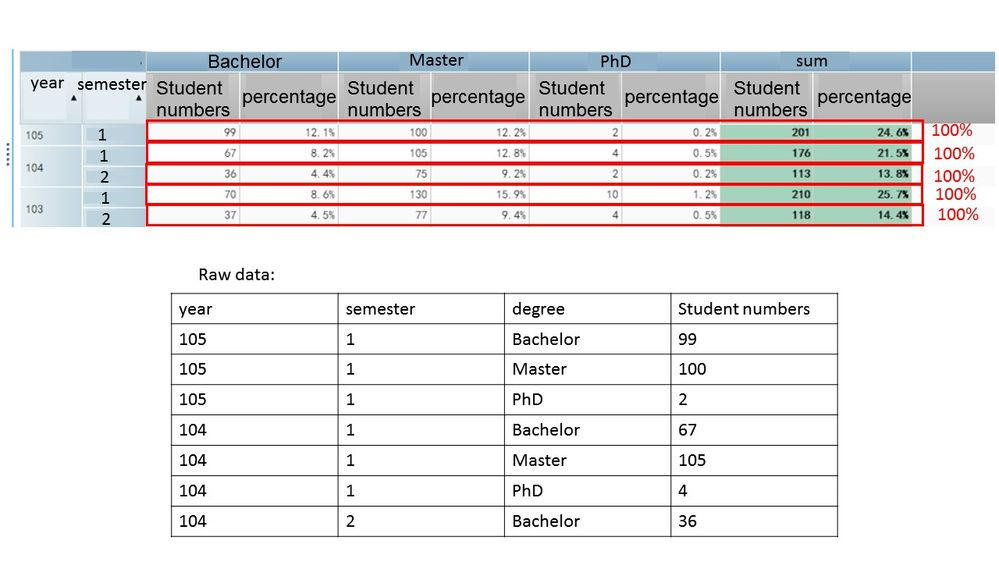## The problem of calculate the percentage

Hi all~

I want to calculate the percentage of each degree student numbers in each year.

but if I use aggregated measure to do this, the results are as follows picture.

how can I do so the result will present like each year's percentage sum for 100%.4 REPLIES 4

## The problem of calculate the percentage

Hi all~

I want to calculate the percentage of each degree student numbers in each year.

but if I use aggregated measure to do this, the results are as follows picture.

how can I do so the result will present like each year's percentage sum for 100%.## Re: The problem of calculate the percentage

What application are you using? SAS, EG, SAS Studio, WRS?

I don't recognize the interface

## Re: The problem of calculate the percentage

sorry ,I should describe it clearly.

Actucally, I use SAS VA.

## Re: The problem of calculate the percentage

I merged the thread into the one in the VA community.

Discussion stats
• 4 replies
• 656 views
• 0 likes
• 3 in conversation